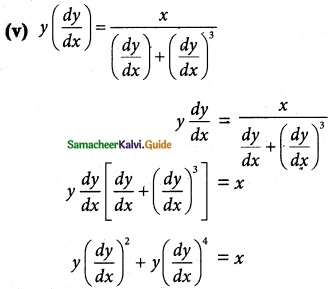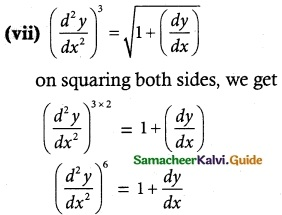Tamilnadu State Board New Syllabus Samacheer Kalvi 12th Maths Guide Pdf Chapter 10 Ordinary Differential Equations Ex 10.1 Textbook Questions and Answers, Notes.

## Tamilnadu Samacheer Kalvi 12th Maths Solutions Chapter 10 Ordinary Differential Equations Ex 10.1

Question 1.
For each of the following equations, determine its order, degree (if exists)
(i) $$\frac { dy }{ dx }$$ + xy = cot x
(ii) ($$\frac { d^3y }{ dx^3 }$$)2/3 – 3 $$\frac { d^2y }{ dx^2 }$$ + 5$$\frac { dy }{ dx }$$ + 4 = 0
(iii) ($$\frac { d^2y }{ dx^2 }$$)2 + ($$\frac { dy }{ dx }$$)² = x sin ($$\frac { d^2y }{ dx^2 }$$)
(iv) $$\sqrt{\frac { dy }{ dx }}$$ – 4 $$\frac { dy }{ dx }$$ – 7x = 0
(v) y($$\frac { dy }{ dx }$$) = $$\frac { x }{ (\frac { dy }{ dx })+(\frac { dy }{ dx })^3 }$$
(vi) x²$$\frac { d^2y }{ dx^2 }$$ + [1 + ($$\frac { dy }{ dx }$$)²]1/2 = 0
(vii) ($$\frac { d^2y }{ dx^2 }$$)³ = $$\sqrt{1+(\frac { dy }{ dx })}$$
(viii) $$\frac { d^2y }{ dx^2 }$$ = xy + cos ($$\frac { dy }{ dx }$$)
(ix) $$\frac { d^2y }{ dx^2 }$$ + 5 $$\frac { dy }{ dx }$$ + ∫ ydx = x³
(x) x = exy($$\frac { dy }{ dx }$$)
Solution:
(i) $$\frac { dy }{ dx }$$ + xy = cot x
In the given equation, the highest order derivative is $$\frac { dy }{ dx }$$ only its power is 1
∴ Its order = 1 & degree = 1(ii) ($$\frac { d^3y }{ dx^3 }$$)2/3 – 3 $$\frac { d^2y }{ dx^2 }$$ + 5$$\frac { dy }{ dx }$$ + 4 = 0
Taking power 3 on both sides, we get
($$\frac { d^3y }{ dx^3 }$$)2 = (3 $$\frac { d^2y }{ dx^2 }$$ – 5$$\frac { dy }{ dx }$$ – 4)³
In the equation (1), the highest order derivative is $$\frac { d^3y }{ dx^3 }$$ and its power is 2.
∴ Its order = 3 & degree = 2

(iii) ($$\frac { d^2y }{ dx^2 }$$)2 + ($$\frac { dy }{ dx }$$)² = x sin ($$\frac { d^2y }{ dx^2 }$$)
In the equation, the highest order derivative is $$\frac { d^2y }{ dx^2 }$$ and its order is 2.
It has a term sin ($$\frac { d^2y }{ dx^2 }$$), so its degree is not defined or degree does not exist.on squaring both sides,
$$\frac { dy }{ dx }$$ = 16 ($$\frac { dy }{ dx }$$)² + 49 x² + 56x $$\frac { dy }{ dx }$$
clearly, it is a differential equation of order = 1 & degree = 2.In this equation, the highest order derivative is $$\frac { dy }{ dx }$$ & its power is 4.
∴ Its order = 1 & degree = 4In this equation, the highest order derivative is $$\frac { d^2y }{ dx^2 }$$ & its power is 2.
∴ Its order = 2 & degree = 2In this equation, the highest order derivative is $$\frac { d^2y }{ dx^2 }$$ & its power is 6.
∴ Its order = 2 & degree = 6(viii) $$\frac { d^2y }{ dx^2 }$$ = xy + cos ($$\frac { dy }{ dx }$$)
In this equation, the highest order derivative is $$\frac { d^2y }{ dx^2 }$$ & its power is 2.
It has a term cos($$\frac { dy }{ dx }$$), so its degree is not defined or degree does not exist.

(ix) $$\frac { d^2y }{ dx^2 }$$ + 5 $$\frac { dy }{ dx }$$ + ∫ ydx = x³
differentiating with respect to x, we get
$$\frac { d^3y }{ dx^3 }$$ + 5 $$\frac { d^2y }{ dx^2 }$$ + y = 3x²
In this equation the highest order derivative is $$\frac { d^3y }{ dx^3 }$$ & its power is 1
∴ Its order = 3 & degree = 1

(x) x = exy($$\frac { dy }{ dx }$$)
In this equation the highest order derivative is $$\frac { dy }{ dx }$$ & its order is 1
It has the term exy($$\frac { dy }{ dx }$$)
So its degree is not defined or degree does not exist.